# A Current Carrying Conductor within a Magnetic Field

Oersted had established that a compass needle gets deflected in the vicinity of a current carrying conductor i.e. this conductor exerts a force on the compass needle. Later on, in the year 1821, Michael Faraday discovered that a current carrying conductor also gets deflected when it is placed in a magnetic field. This can be said that magnetic field and this current carrying conductor exert a force on each other in their vicinity.
Suppose a conductor carries current I and it is with the length (l). As it is carrying current (DC), some flux lines will be generated around the conductor and they are concentric with the central axis of the conductor. So an electromagnetic field is established due to this current through this conductor.

Following right hand thumb rule the magnetic flux lines get the direction along the bent fingers when thumb denotes the direction of the current flow, i.e. shown in the figure below.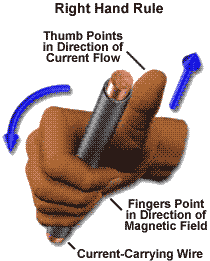This current carrying conductor is placed between two poles of a horse shoe magnet of flux density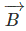. This magnet is tightly fixed to the ground. Conductor is not fixed, rather it is free to move. The length of the conductor is just perpendicular of the permanent magnetic field of the horse shoe.

So, it is clear that the direction of current and magnetic field is normal to each other.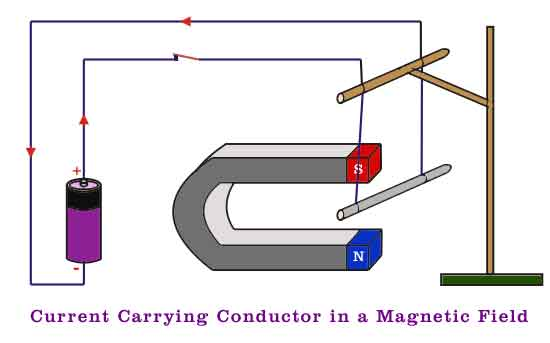Now two magnetic fields (electromagnetic field by the conductor and permanent magnetic field by the horse shoe magnet) are in their action.The concentric circles of electromagnetic flux due to flowing current (I) through this conductor try to repel the magnetic flux of the permanent magnet at that situation.

Let us consider the force is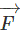.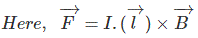Here the direction of the current depends on the orientation of length of the current carrying conductor (l), so vector is taken for length only. The forceis the cross product of length vector (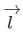) and the flux density vector (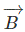). Now,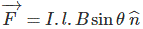Here, θ is the angle between two vectors and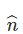is the unit vector of the force in the perpendicular direction with respect to two vectors direction.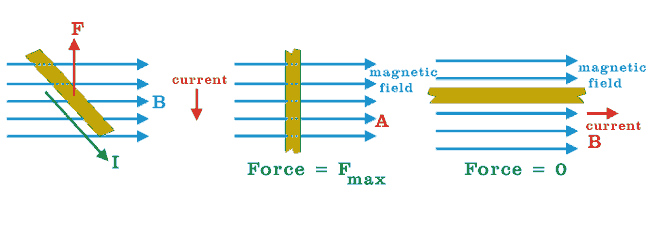In This direction of force the conductor will move to. This consequent can be simplified with an easy rule, i.e. Fleming’s Left Hand rule. By stretching three fingers of left hand in perpendicular manner with each other, if the direction of the current is denoted by middle finger of the left hand and the second finger is for direction of the magnetic flux then the thumb of the left hand denotes the direction of the conductor’s movement.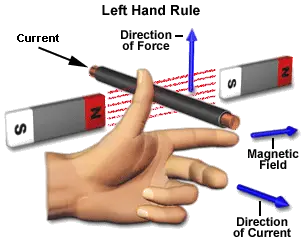Now the direction of the current through this conductor depends on the conductor in which orientation the conductor is placed between two poles of the magnet. So the current carrying conductor always faces a force in the vicinity of a permanent magnet or any electro-magnet. Based on this phenomenon DC motor rotates.

Want To Learn Faster? 🎓
Get electrical articles delivered to your inbox every week.
No credit card required—it’s 100% free.